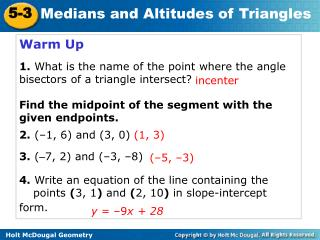# Warm Up 1. What is the name of the point where the angle bisectors of a triangle intersect? - PowerPoint PPT PresentationDownload PresentationWarm Up 1. What is the name of the point where the angle bisectors of a triangle intersect?

Warm Up 1. What is the name of the point where the angle bisectors of a triangle intersect?Download Presentation## Warm Up 1. What is the name of the point where the angle bisectors of a triangle intersect?

- - - - - - - - - - - - - - - - - - - - - - - - - - - E N D - - - - - - - - - - - - - - - - - - - - - - - - - - -
##### Presentation Transcript

1. Warm Up 1.What is the name of the point where the angle bisectors of a triangle intersect? Find the midpoint of the segment with the given endpoints. 2. (–1, 6) and (3, 0) 3. (–7, 2) and (–3, –8) 4. Write an equation of the line containing the points (3, 1) and (2, 10) in slope-intercept form. incenter (1, 3) (–5, –3) y = –9x + 28

2. Learning Targets I will apply properties of medians and altitudes of a triangle.

3. Vocabulary median of a triangle centroid of a triangle altitude of a triangle orthocenter of a triangle

4. A median of a triangleis a segment whose endpoints are a vertex of the triangle and the midpoint of the opposite side. Every triangle has three medians, and the medians are concurrent.

5. The point of concurrency of the medians of a triangle is the centroid of the triangle. The centroid is always inside the triangle. The centroid is also called the center of gravity because it is the point where a triangular region will balance.

6. Practice Pg 329, #3 - 6 3. VW = 136 4. WX = 68 5. RY = 156 6. WY = 52

7. Example 2: Problem-Solving Application A sculptor is shaping a triangular piece of iron that will balance on the point of a cone. At what coordinates will the triangular region balance?

8. 1 Make a Plan Understand the Problem 2 Example 2 Continued The answer will be the coordinates of the centroid of the triangle. The important information is the location of the vertices, A(6, 6), B(10, 7), and C(8, 2). The centroid of the triangle is the point of intersection of the three medians. So write the equations for two medians and find their point of intersection.

9. 3 Solve Let M be the midpoint of AB and N be the midpoint of AC. CM is vertical. Its equation is x = 8. BN has slope 1. Its equation is y = x – 3. The coordinates of the centroid are D(8, 5). Example 2 Continued

10. Let L be the midpoint of BC. The equation for AL is , which intersects x = 8 at D(8, 5). 4 Example 2 Continued Look Back

11. An altitude of a triangle is a perpendicular segment from a vertex to the line containing the opposite side. Every triangle has three altitudes. An altitude can be inside the triangle, outside the triangle, or on the triangle, depending on what kind of a triangle it is.

12. In ΔQRS, altitude QY is inside the triangle, but RX and SZ are not. Notice that the lines containing the altitudes are concurrent at P. This point of concurrency is the orthocenter of the triangle.

13. Helpful Hint The height of a triangle is the length of an altitude.

14. Practice Pg 329, #8 - 10 8. (4, -1) 9. (2, -3) 10. (-5, -4) Homework: Pg 330, #12 – 26, 29 - 32